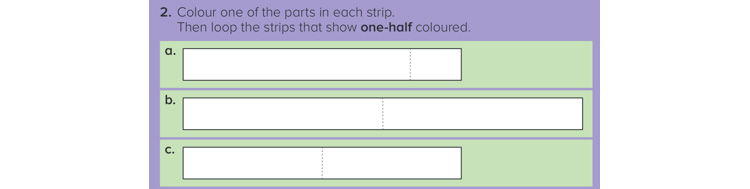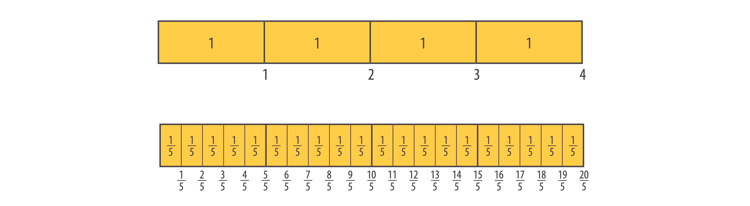# Using multiple fraction representations to build a foundation of success in mathematics

What is the ‘full’ range of materials we need to teach common fractions?James Burnett, Senior Author

The teaching of fractions is often identified as the point in primary school instruction when the “wheels tend to fall off’. While there could be many reasons for this, the overuse of one ‘preferred’ resource (e.g., circular discs) at the expense of a range of representations will often create gaps in understanding at best, and misconceptions at its worst. The work of Zoltan Dienes, the Hungarian mathematician and learning theorist described multiple embodiments. He promoted the use of the full range of resources that collectively show the many features of a single mathematics concept. This raises the question: what is the ‘full’ range of materials we need to teach common fractions?

There are essentially four different representations of common fractions that students should progressively encounter: length, area, set and number line models. All of these are pre-requisites to the many applications that use fraction knowledge in secondary school.

The Length Model
The earliest experiences students have when learning fractions are hands-on activities involving paper folding and the colouring of 2D shapes such as those below. Experiences such as these enable students to interpret fractions as being part of whole (whole to part thinking).(©ORIGO Stepping Stones: Reproduced with permission)

Length models are those which show one-directional cuts or folds. This attribute enables it to be extended to show fractions greater than one. The two strips below show four whole ones. Each of the wholes has then been divided into fifths. This image is ideal for proper and improper fractions as well as linking to mixed numbers.The length model is also perfect for addition and subtraction of fractions. Students can shade the strip to show the sum of two or more fractions and easily work with totals that are greater than one.

The Area Model
Paper folding and colouring can also be used with area models. These representations are generally considered to be those which show multi-directional cuts or folds like those shown below.As with length models, the size and shape of the whole can vary. Experiences like these that require students to identify and colour ¼ serve to develop ‘whole to part’ thinking.

Plastic fraction discs can also be found in schools. Each disc is generally of one colour and often pre-cut into multiple parts. When students pick up one part, they are left wondering about the size of the whole. These resources develop the ‘part to whole’ thinking which is commonly overlooked.

As we move up the grades, the area model is again useful for developing the idea of equivalent fractions (think paper folding) and is essential for teaching multiplication and division of fractions when the work is all less than one. But it is less useful for showing improper fractions. For example, how do we show 1 ¾? We need more wholes, which then requires us to clearly define the size of the whole. This increases the level of complexity which some students find challenging.

The Set Model
The set model is the only representation based on discrete countable objects. Most students enter school with a good understanding of equal shares. For example, if they are asked to share the eggs below between two people, they usually say that each group of six eggs is one-half of the total. However, without the ‘frame’ (carton) it is difficult to determine the size of the whole.One of the most useful applications of the set representation involves fractions as operators (1/2 of 120 or ½ x 120). In this case, students will benefit from linking ½ x 120 to 120 ÷ 2. Also, finding the percentage of an amount of money involves the set model (25% of \$200 is the same as ¼ of \$200 – assuming they have had prior experience with connecting 25% to a fraction form). Use the set model where it is the most beneficial without leading to misconceptions.

The Number Line Model
The number line is closely related to the length model. Fractions on this model are described as a distance from 0. While international convention defines the whole as always being the distance from 0 to 1, it is still best to establish the whole for students. In this picture, the thirds can only be determined after 1 has been identified on the number line.The number line provides the clearest picture linking fractions to whole numbers and shows the meaning of proper and improper fractions, and mixed numbers. Like the length model, the number line is good for establishing equivalence and for addition and subtraction of fractions. The number line is an abstract mathematical tool so students will, at first, lack everyday experiences with this valuable resource. It is always useful to work with the length model first and then transition students to the number line.

Conclusion
There are four distinct models of fractions that students need to encounter at various years of schooling. Despite their utility, visual models do not automatically guarantee success. Care needs to be taken when selecting an appropriate model based on the prior experiences of the students with fractions. It is also important to consider the fraction content that is being taught and use the model that will clearly display the desired ideas.